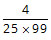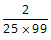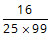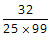# GMAT Quant - Number Theory & Number Properties

Must solve GMAT practice questions in Number Theory and Number Properties. A good number of questions appear from this topic. It is a core topic. Includes concepts such as LCM, HCF, Factors, number of factors, reminders, divisors, factorial, prime numbers, composite numbers, relatively prime numbers. Focus on understanding the basics and principles behind each of the concepts. In short, it is not just sufficient to know how to find the LCM of two or more numbers - you also need to know what LCM actually means and in what scenario will one find the LCM of numbers.

The categorization of the type of question and the concept tested are listed in the right column for each question. Detailed explanatory answers, solution videos and slide decks are also provided.

• 1
• If two distinct integers a and b are picked from {1, 2, 3, 4....100} and multiplied, what is the probability that the resulting number has EXACTLY 3 factors? A.B.C.D.E.• Problem Solving
• Discrete Probability
• Number of factors
The required probability isSolution

• 2
• What is the remainder when the positive integer x is divided by 6? Statement 1: When x is divided by 7, the remainder is 5.
Statement 2: When x is divided by 9, the remainder is 3.
• Data Sufficiency
• Arithmetic
• Remainders